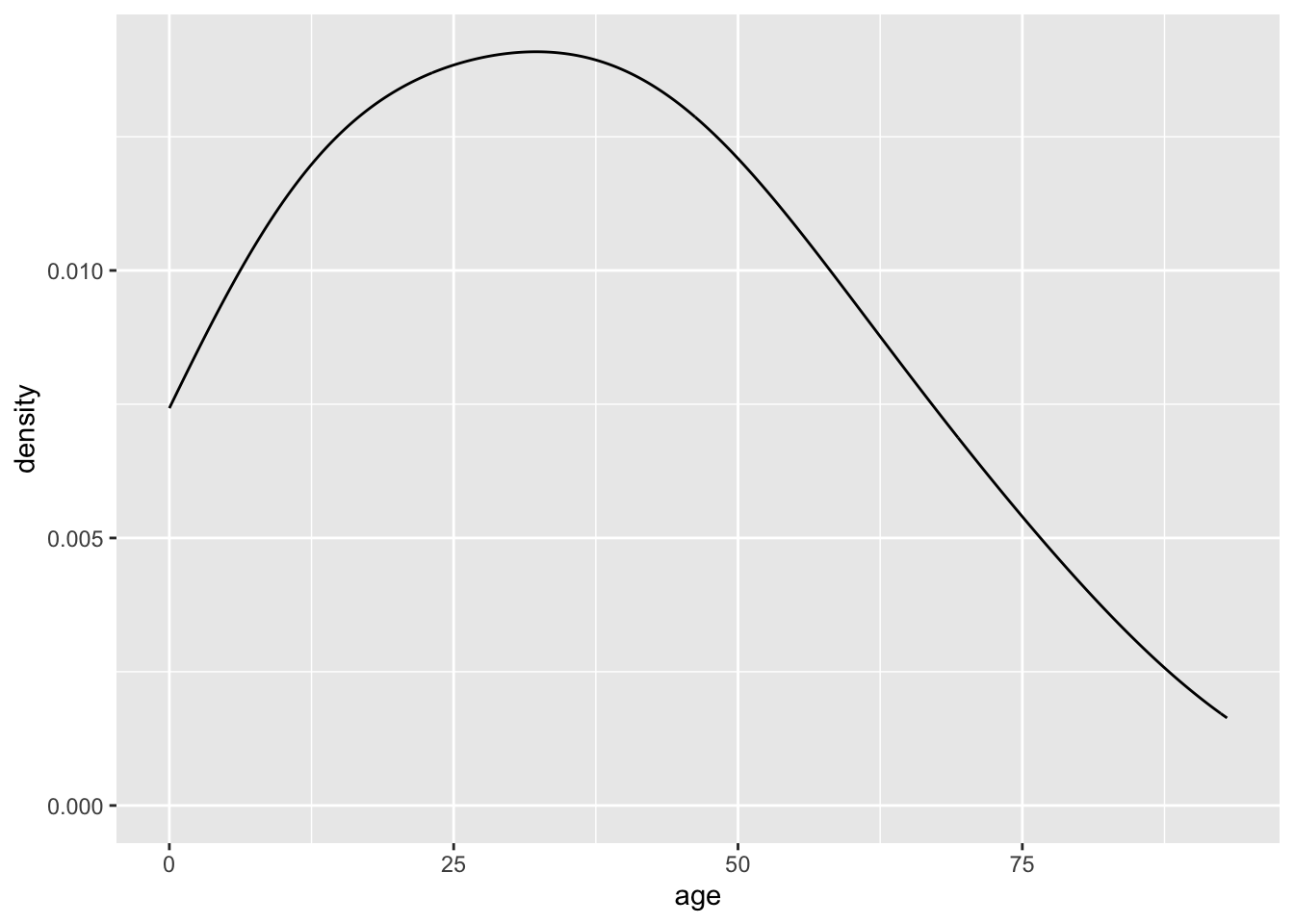# 4 One Variable

If you are starting from this page, please run the code at Libraries and Data Setup before proceeding.

## 4.1 Discrete

### 4.1.1 Barplots

To understand the distribution of a discrete variable (a variable with a limited number of values or categories), we often want to know how the count of each category, that is, the number of observations at each level of the variable.

A barplot is useful for visualizing counts, and `geom_bar()` gives the count of each category by default (`stat = "count"`).

``````ggplot(acs, aes(x = edu)) +
geom_bar()``````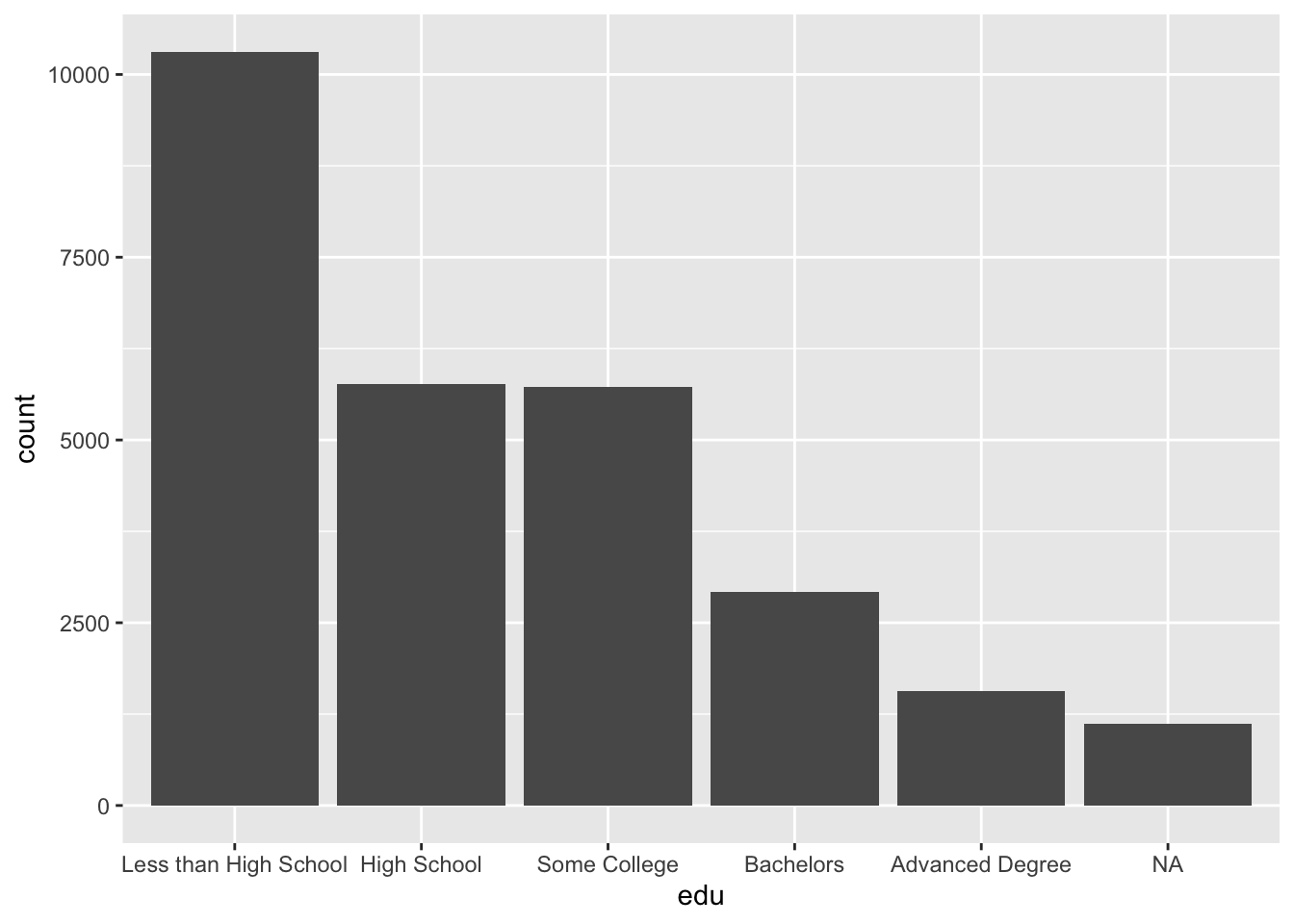The above plot includes missing values (`NA`), which we can drop by making use of `filter()` and `is.na()`.

Note that, while functions outside ggplot are linked with the pipe operator `%>%`, ggplot elements are joined with the addition operator `+`.

ggplot has no separate function for horizontal barplots. Instead, supply the `coord_flip()` function to flip the horizontal and vertical coordinates, so that our `x` aesthetic is shown along the vertical axis.

``````acs %>%
filter(!is.na(edu)) %>%
ggplot(aes(x = edu)) +
geom_bar() +
coord_flip()``````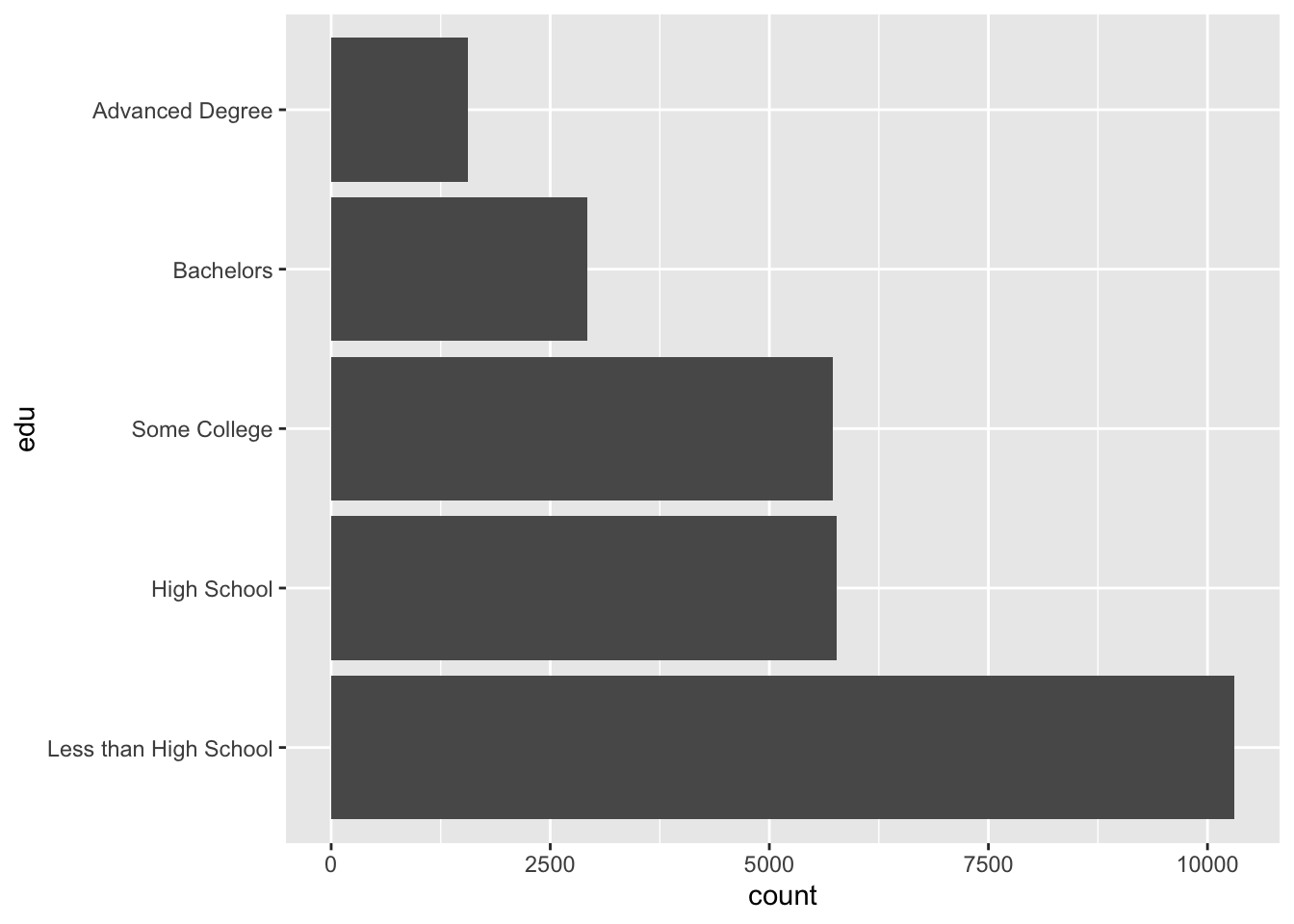## 4.2 Continuous

### 4.2.1 Histograms

The standard histogram displays counts along a continuous variable, which is divided into a number of bins. The default number of bins in `geom_histogram()` is 30. With an age range of [0, 93], each bin is about 93 / 30 = 3.1 years wide.

A histogram that has been divided into discrete bins, or categories, is actually a barplot. In the barplots above, a continuous education variable was already divided into five “bins” of unequal width, something like 0-11 years of education (“Less than High School”), 12 years (“High School”), 13-15 years (“Some College”), 16 years (“Bachelors”), and 17+ years (“Advanced Degree”). In the histogram below, we divide our continuous age variable into 30 categories of equal width.

``````ggplot(acs, aes(x = age)) +
geom_histogram()``````
``## `stat_bin()` using `bins = 30`. Pick better value with `binwidth`.``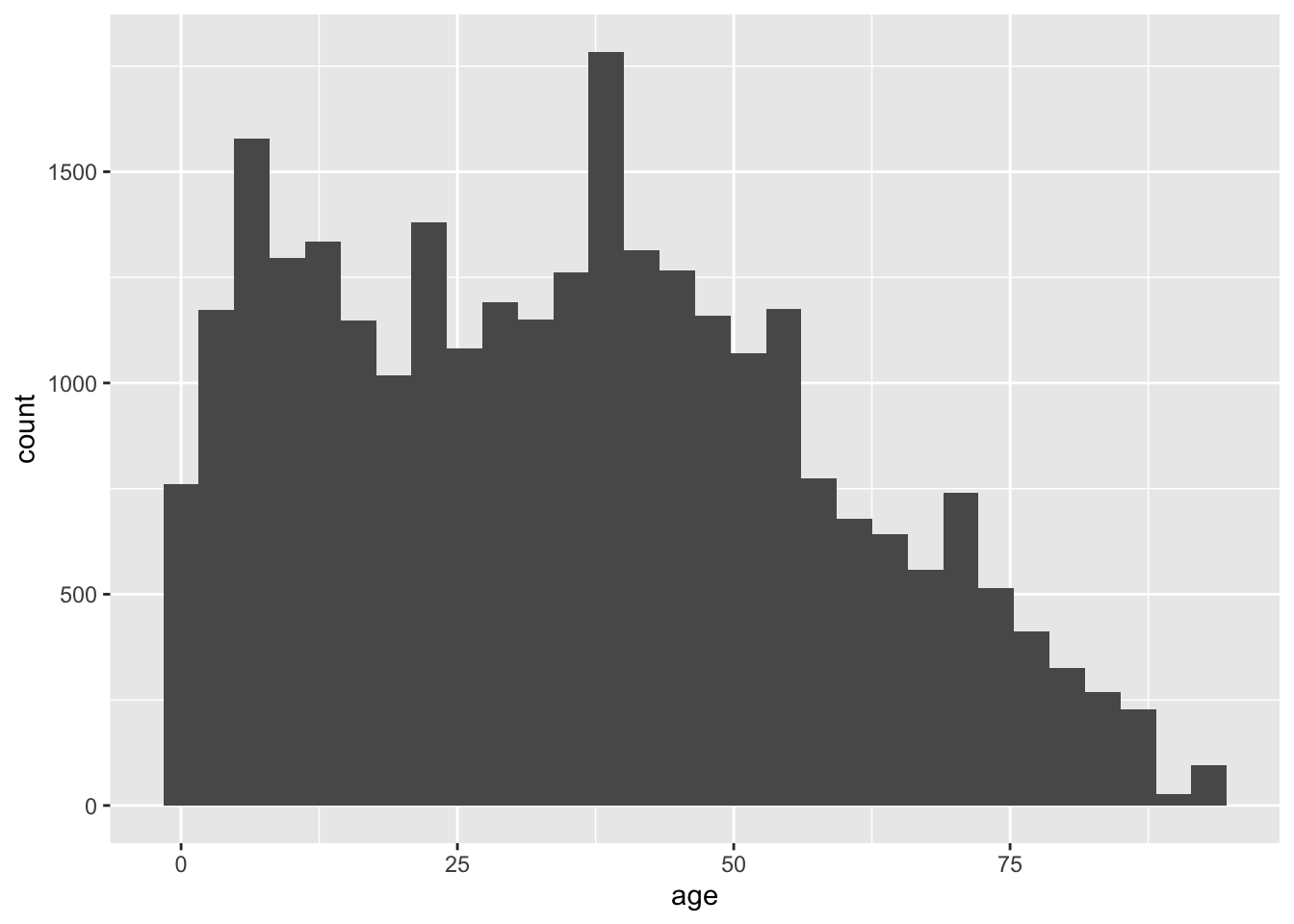The width of each bin can be modified with the `binwidth` argument of `geom_histogram()`. We can set it to 10 so that the first bin is 0-10 years old, the second is 10-20, and so on.

``````ggplot(acs, aes(x = age)) +
geom_histogram(binwidth = 10)``````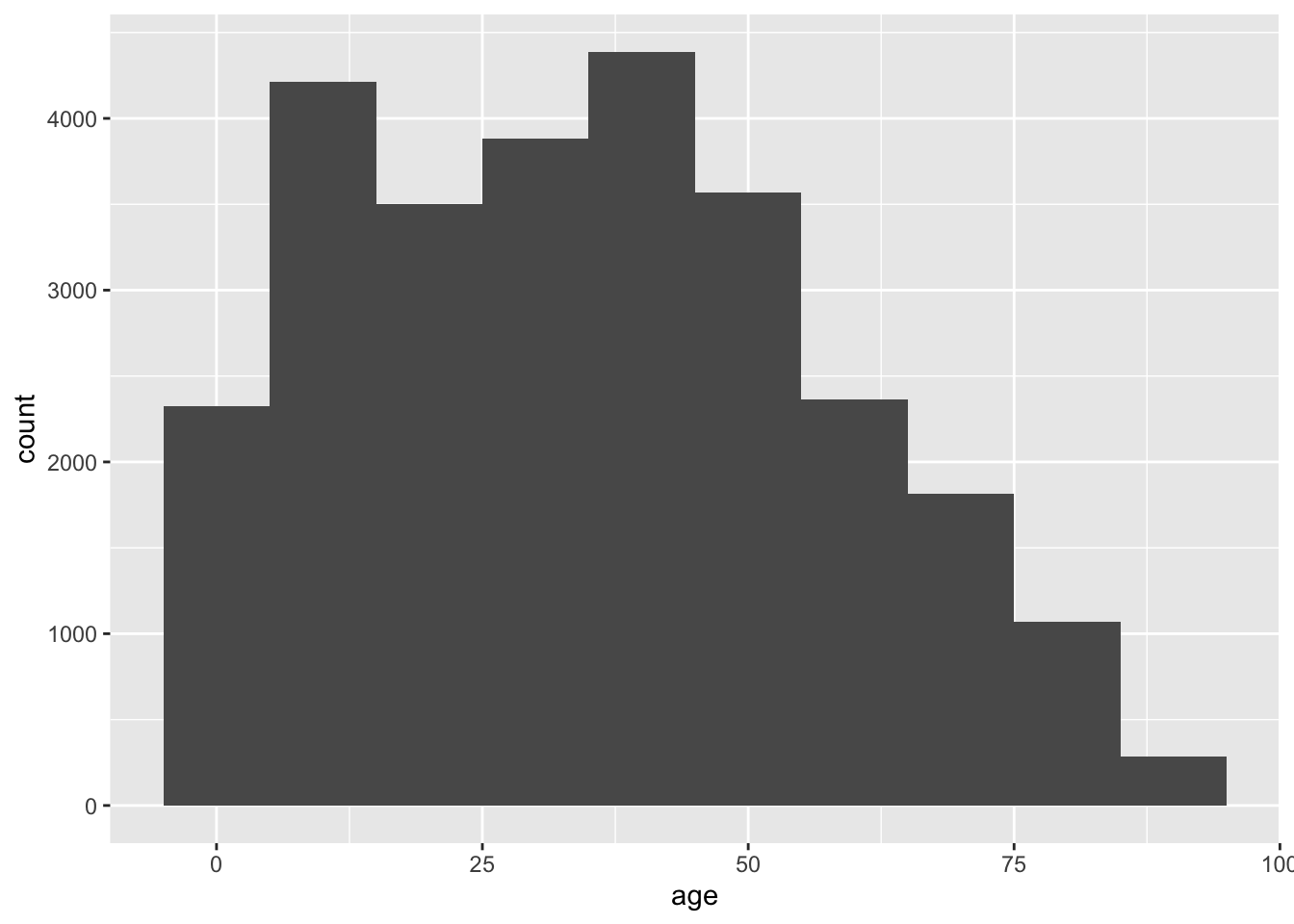In the above plot, we lost a fair amount of information from the first histogram. The decrease in counts by age appears monotone after 50, but we know from the first histogram that there is a concentration of values (a peak) around 70.

Change `binwidth` to 1 for narrower bins, and so increase the amount of information in the plot.

``````ggplot(acs, aes(x = age)) +
geom_histogram(binwidth = 1)``````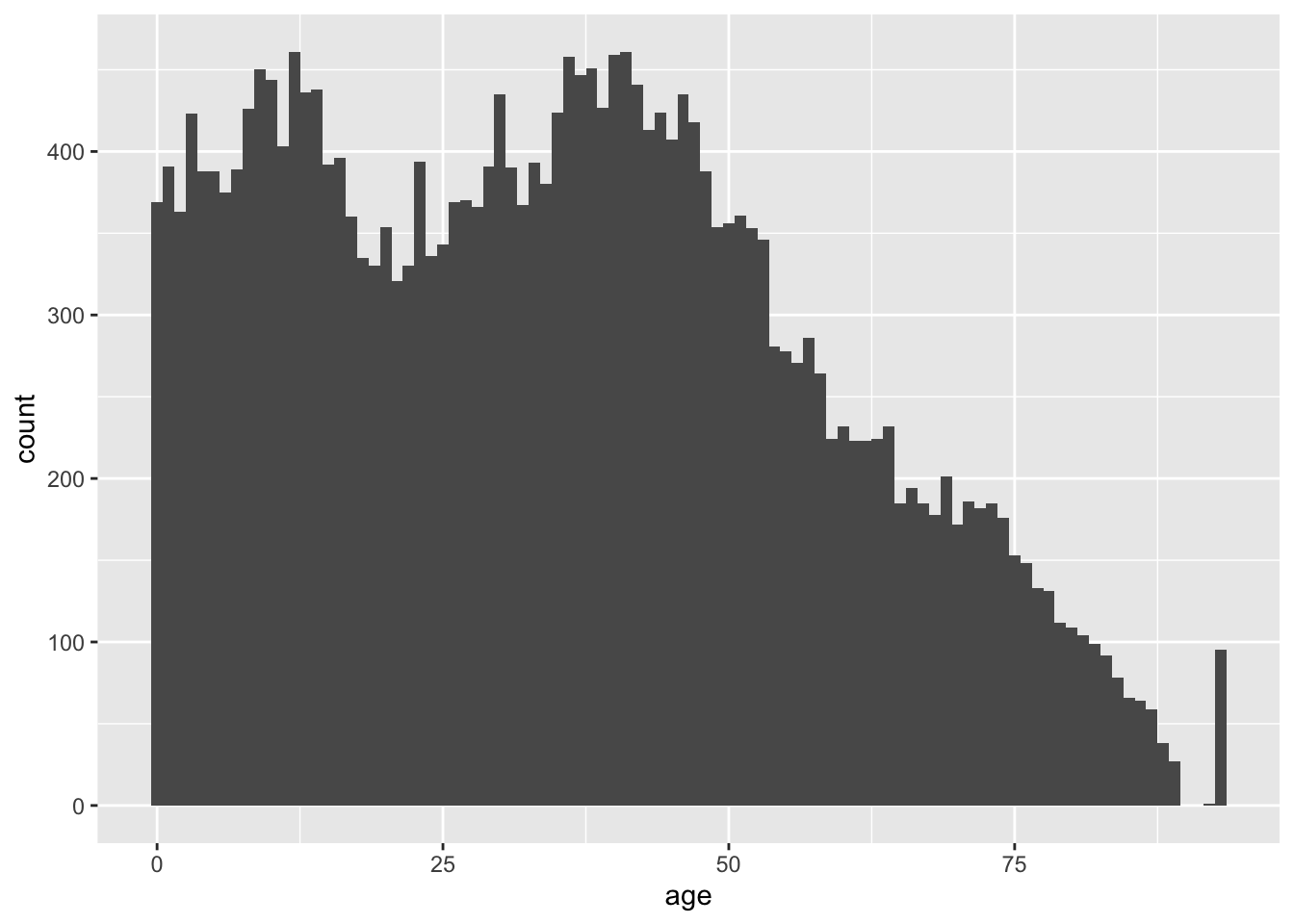We now have the opposite problem as the previous plot. We have too much information, and we may be tempted to over-interpret fine differences in counts across adjacent values in age. We may choose to revert to the default of 30 bins for this variable.

Also, notice the relatively tall bar at the far right of the plot. Either age is top-coded somewhere in the 90s, or something very strange is happening in our sample.

### 4.2.2 Density Plots

Another choice for a single continous variable is a smoothed density plot, which can be created with `geom_density()`.

``````ggplot(acs, aes(x = age)) +
geom_density()``````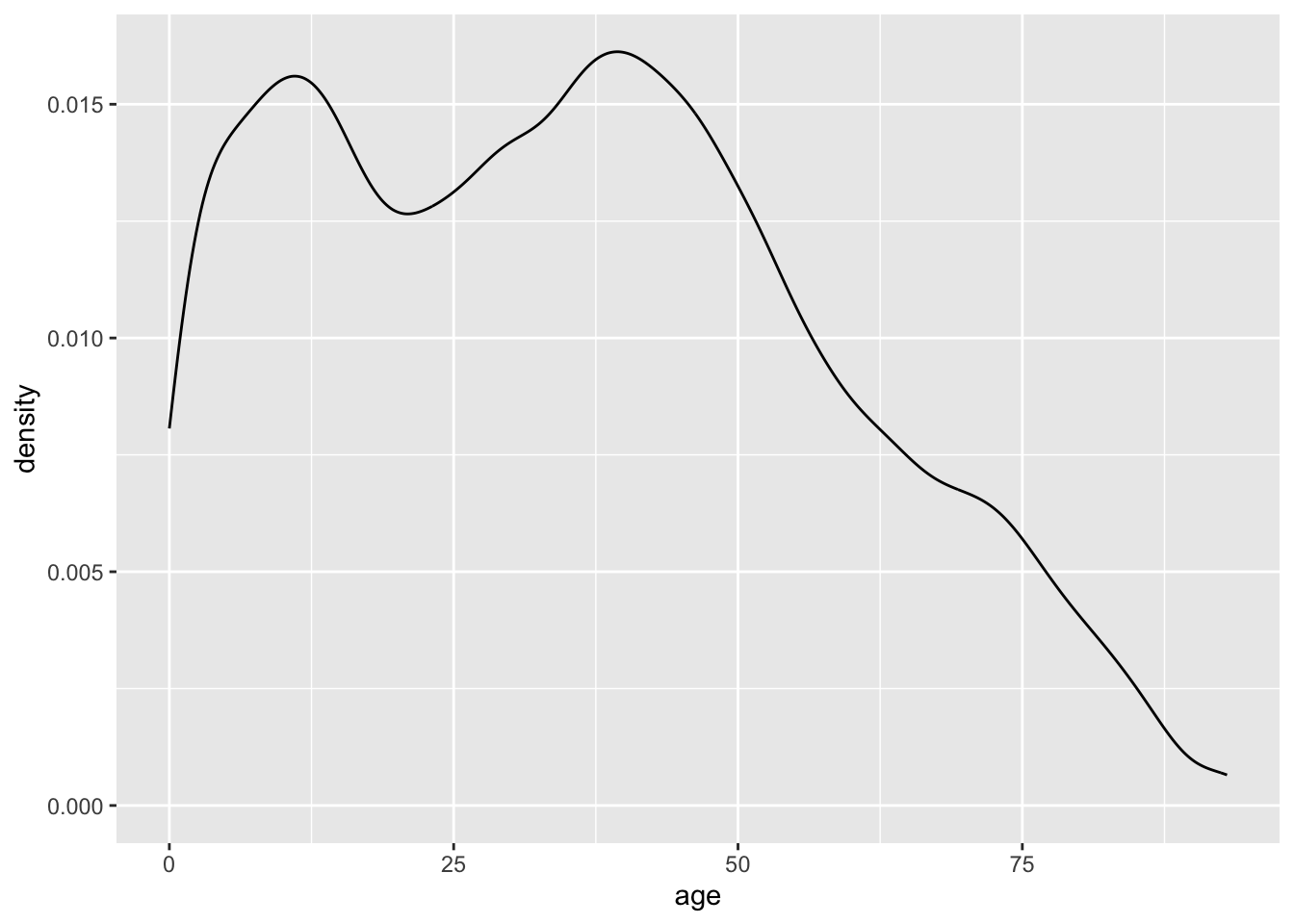The height of the density plot is scaled so that the total area under the curve is equal to one, so the values on the y-axis have no practical meaning.

Just like how we adjusted the binwidth of our histograms, we can also adjust the granularity of density plots.

The bandwidth can be selected directly with the `bw` argument, but it may be easier to supply the `adjust` argument with a constant, which ggplot will multiple against the bandwidth.

A smaller bandwidth makes a more jagged plot. Too small of a bandwidth, and the density plot starts to look like a histogram.

``````ggplot(acs, aes(x = age)) +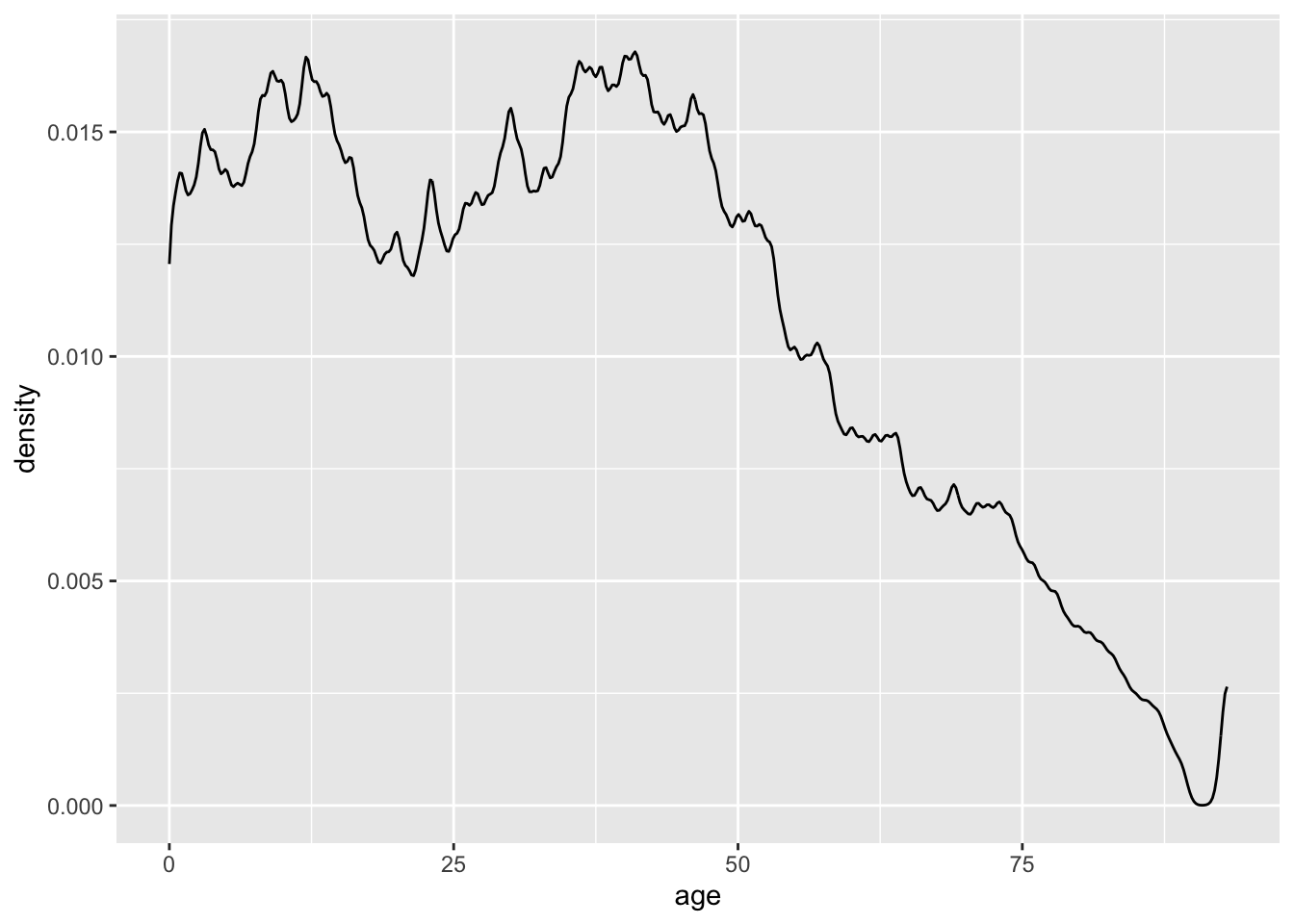``````ggplot(acs, aes(x = age)) +